# circle segment

This calculator considers the parameters of a circle segment, namely:

• arc length (L),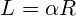• chord length (C),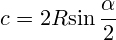• area (S),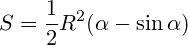• height (h),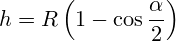Here 2 calculator to calculate the parameters of the segment:

1) segment of a circle is solved by a radius (R) and angle (A).

2) find the circle segment by means of height and chord length.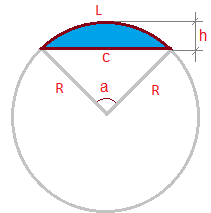﻿

Segment
Length chords:
Height:
The perimeter:
Length arc:
Area:

However, as true noticed our the user:"on practice hourit it happens, what as radius arc, so and angle unknown" (cm. length arc ). For this case for calculation square segment and length arc can use the following calculator:
Settings segment at the chord and height
Area:
Length arc:
Angle (C):
The perimeter:

Calculator calculates radius circle at length chords and height segment at the following the formula:

Further knowing radius and length chords, easy find angle segment at the formula:

The rest settings segment, calculated similarly first calculator, at formulas, given in the beginning article.

The following calculator calculates area segment at height and radius:
Area segment circle at radius and height
Area:
Length chords:
The perimeter:
Length arc:
Angle (C):